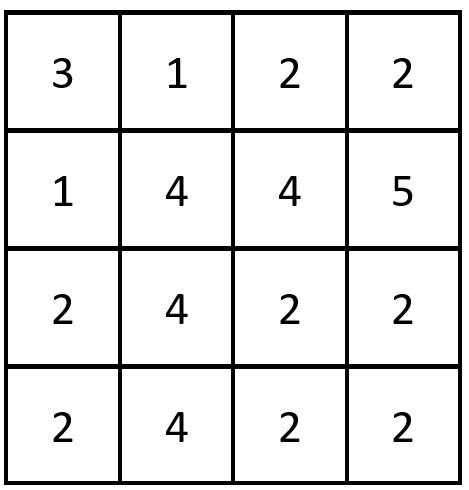2352. Equal Row and Column Pairs

#### QUESTION:

Given a 0-indexed n x n integer matrix grid, return the number of pairs (ri, cj) such that row ri and column cj are equal.

A row and column pair is considered equal if they contain the same elements in the same order (i.e., an equal array).

Example 1:``````Input: grid = [[3,2,1],[1,7,6],[2,7,7]]
Output: 1
Explanation: There is 1 equal row and column pair:
- (Row 2, Column 1): [2,7,7]
``````

Example 2:``````Input: grid = [[3,1,2,2],[1,4,4,5],[2,4,2,2],[2,4,2,2]]
Output: 3
Explanation: There are 3 equal row and column pairs:
- (Row 0, Column 0): [3,1,2,2]
- (Row 2, Column 2): [2,4,2,2]
- (Row 3, Column 2): [2,4,2,2]
``````

Constraints:

``````n == grid.length == grid[i].length
1 <= n <= 200
1 <= grid[i][j] <= 105
``````

#### SOLUTION:

``````class Solution {
func equalPairs(_ grid: [[Int]]) -> Int {
var colum: [[Int]] = []
for i in 0...grid.count-1 {
var tmpArr: [Int] = []
for j in 0...grid.count-1 {
tmpArr.append(grid[j][i])
}
colum.append(tmpArr)
}
var result = 0
for gr in grid {
for co in colum {
if gr == co {
result += 1
}
}
}
return result
}
}

``````